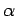# 4.5: Alpha Decay

$$\newcommand{\vecs}{\overset { \rightharpoonup} {\mathbf{#1}} }$$ $$\newcommand{\vecd}{\overset{-\!-\!\rightharpoonup}{\vphantom{a}\smash {#1}}}$$$$\newcommand{\id}{\mathrm{id}}$$ $$\newcommand{\Span}{\mathrm{span}}$$ $$\newcommand{\kernel}{\mathrm{null}\,}$$ $$\newcommand{\range}{\mathrm{range}\,}$$ $$\newcommand{\RealPart}{\mathrm{Re}}$$ $$\newcommand{\ImaginaryPart}{\mathrm{Im}}$$ $$\newcommand{\Argument}{\mathrm{Arg}}$$ $$\newcommand{\norm}{\| #1 \|}$$ $$\newcommand{\inner}{\langle #1, #2 \rangle}$$ $$\newcommand{\Span}{\mathrm{span}}$$ $$\newcommand{\id}{\mathrm{id}}$$ $$\newcommand{\Span}{\mathrm{span}}$$ $$\newcommand{\kernel}{\mathrm{null}\,}$$ $$\newcommand{\range}{\mathrm{range}\,}$$ $$\newcommand{\RealPart}{\mathrm{Re}}$$ $$\newcommand{\ImaginaryPart}{\mathrm{Im}}$$ $$\newcommand{\Argument}{\mathrm{Arg}}$$ $$\newcommand{\norm}{\| #1 \|}$$ $$\newcommand{\inner}{\langle #1, #2 \rangle}$$ $$\newcommand{\Span}{\mathrm{span}}$$$$\newcommand{\AA}{\unicode[.8,0]{x212B}}$$

Many types of heavy atomic nucleus spontaneously decay to produce daughter nucleii via the emission of $$\alpha$$-particles (i.e., helium nucleii) of some characteristic energy. This process is know as $$\alpha$$-decay. Let us investigate the $$\alpha$$-decay of a particular type of atomic nucleus of radius $$R$$, charge-number $$Z$$, and mass-number $$A$$. Such a nucleus thus decays to produce a daughter nucleus of charge-number $$Z_1=Z-2$$ and mass-number $$A_1=A-4$$, and an $$\alpha$$-particle of charge-number $$Z_2=2$$ and mass-number $$A_2=4$$. Let the characteristic energy of the $$\alpha$$-particle be $$E$$. Incidentally, nuclear radii are found to satisfy the empirical formula $R = 1.5\times 10^{-15}\,A^{1/3}\,{\rm m}=2.0\times 10^{-15}\,Z_1^{\,1/3}\,{\rm m}$ for $$Z\gg 1$$.

In 1928, George Gamow proposed a very successful theory of $$\alpha$$-decay, according to which the $$\alpha$$-particle moves freely inside the nucleus, and is emitted after tunneling through the potential barrier between itself and the daughter nucleus . In other words, the $$\alpha$$-particle, whose energy is $$E$$, is trapped in a potential well of radius $$R$$ by the potential barrier $V(r) = \frac{Z_1\,Z_2\,e^{\,2}}{4\pi\,\epsilon_0\,r}$ for $$r>R$$.

Making use of the WKB approximation (and neglecting the fact that $$r$$ is a radial, rather than a Cartesian, coordinate), the probability of the $$\alpha$$-particle tunneling through the barrier is $|T|^{\,2} = \exp\left(-\frac{2\sqrt{2\,m}}{\hbar}\int_{r_1}^{r_2} \sqrt{V(r)-E}\,dr\right),$ where $$r_1=R$$ and $$r_2 = Z_1\,Z_2\,e^{\,2}/(4\pi\,\epsilon_0\,E)$$. Here, $$m=4\,m_p$$ is the $$\alpha$$-particle mass. The previous expression reduces to $|T|^{\,2} = \exp\left(-2\sqrt{2}\,\beta \int_{1}^{E_c/E}\left[\frac{1}{y}-\frac{E}{E_c}\right]^{1/2} dy\right),$ where $\beta = \left(\frac{Z_1\,Z_2\,e^{\,2}\,m\,R}{4\pi\,\epsilon_0\,\hbar^{\,2}}\right)^{1/2} = 0.74\,Z_1^{\,2/3}$ is a dimensionless constant, and $E_c = \frac{Z_1\,Z_2\,e^{\,2}}{4\pi\,\epsilon_0\,R} = 1.44\,Z_1^{\,2/3}\,\,{\rm MeV}$ is the characteristic energy the $$\alpha$$-particle would need in order to escape from the nucleus without tunneling. Of course, $$E\ll E_c$$. It is easily demonstrated that $\int_1^{1/\epsilon}\left(\frac{1}{y} - \epsilon\right)^{1/2} dy \simeq \frac{\pi}{2\sqrt{\epsilon}}-2$ when $$\epsilon\ll 1$$. Hence. $|T|^{\,2} \simeq \exp\left(-2\sqrt{2}\,\beta\left[\frac{\pi}{2}\sqrt{\frac{E_c}{E}}-2\right]\right).$

Now, the $$\alpha$$-particle moves inside the nucleus with the characteristic velocity $$v= \sqrt{2\,E/m}$$. It follows that the particle bounces backward and forward within the nucleus at the frequency $$\nu\simeq v/R$$, giving $\nu\simeq 2\times 10^{28}\,\,{\rm yr}^{-1}$ for a 1 MeV $$\alpha$$-particle trapped inside a typical heavy nucleus of radius $$10^{-14}$$ m. Thus, the $$\alpha$$-particle effectively attempts to tunnel through the potential barrier $$\nu$$ times a second. If each of these attempts has a probability $$|T|^{\,2}$$ of succeeding then the probability of decay per unit time is $$\nu\,|T|^{\,2}$$. Hence, if there are $$N(t)\gg 1$$ undecayed nuclii at time $$t$$ then there are only $$N+dN$$ at time $$t+dt$$, where $dN = - N\,\nu\,|T|^{\,2}\,dt.$ This expression can be integrated to give $N(t) = N(0)\,\exp(-\nu\,|T|^{\,2}\,t).$ Now, the half-life, $$\tau$$, is defined as the time which must elapse in order for half of the nuclii originally present to decay. It follows from the previous formula that $\tau = \frac{\ln 2}{\nu\,|T|^{\,2}}.$ Note that the half-life is independent of $$N(0)$$.

Finally, making use of the previous results, we obtain $\label{e5.64} \log_{10}[\tau ({\rm yr})] = -C_1 - C_2\,Z_1^{\,2/3} + C_3\,\frac{Z_1}{\sqrt{E({\rm MeV})}},$ where \begin{aligned} C_1 &= 28.5,\\[0.5ex] C_2 &= 1.83,\\[0.5ex] C_3 &= 1.73.\end{aligned}Figure 15: The experimentally determined half-life, $$\begin{equation}\tau_{\mathrm{e} x}\end{equation}$$ of various atomic nucleii which decay viaemission versus the best-fit theoretical half-life $$\begin{equation}\log _{10}\left(\tau_{t h}\right)=-28.9-1.60 Z_{1}^{2 / 3}+1.61 Z_{1} / \sqrt{E}\end{equation}$$. Both half-lives are measured in years. Here, $$\begin{equation}Z_{1}=Z-2\end{equation}$$. Both half-lives are measured in years. Here, $$\begin{equation}Z_{1}=Z-2, \text { where } Z\end{equation}$$ is the charge number of the nucleus, andthe characteristic energy of the emitted-particle in MeV. In order of increasing half-life, the points correspond to the following nucleii: Rn 215, Po 214, Po 216, Po 197, Fm 250, Ac 225, U 230, U 232, U 234, Gd 150, U 236, U 238, Pt 190, Gd 152, Nd 144. Data obtained from IAEA Nuclear Data Centre.

Equation ([e5.64]) is known as the Geiger-Nuttall formula, because it was discovered empirically by H. Geiger and J.M. Nuttall in 1911 .

The half-life, $$\tau$$, the daughter charge-number, $$Z_1=Z-2$$, and the $$\alpha$$-particle energy, $$E$$, for atomic nucleii which undergo $$\alpha$$-decay are indeed found to satisfy a relationship of the form ([e5.64]). The best fit to the data (see Figure [fal]) is obtained using \begin{aligned} C_1 &= 28.9,\\[0.5ex] C_2 &= 1.60,\\[0.5ex] C_3 &= 1.61.\end{aligned} Note that these values are remarkably similar to those calculated previously.

$$\newcommand {\ltapp} {\stackrel {_{\normalsize<}}{_{\normalsize \sim}}}$$ $$\newcommand {\gtapp} {\stackrel {_{\normalsize>}}{_{\normalsize \sim}}}$$ $$\newcommand {\btau}{\mbox{\boldmath\tau}}$$ $$\newcommand {\bmu}{\mbox{\boldmath\mu}}$$ $$\newcommand {\bsigma}{\mbox{\boldmath\sigma}}$$ $$\newcommand {\bOmega}{\mbox{\boldmath\Omega}}$$ $$\newcommand {\bomega}{\mbox{\boldmath\omega}}$$ $$\newcommand {\bepsilon}{\mbox{\boldmath\epsilon}}$$